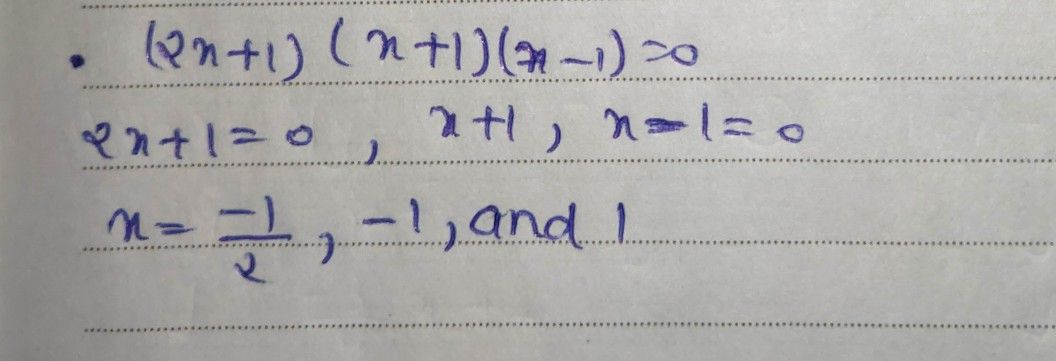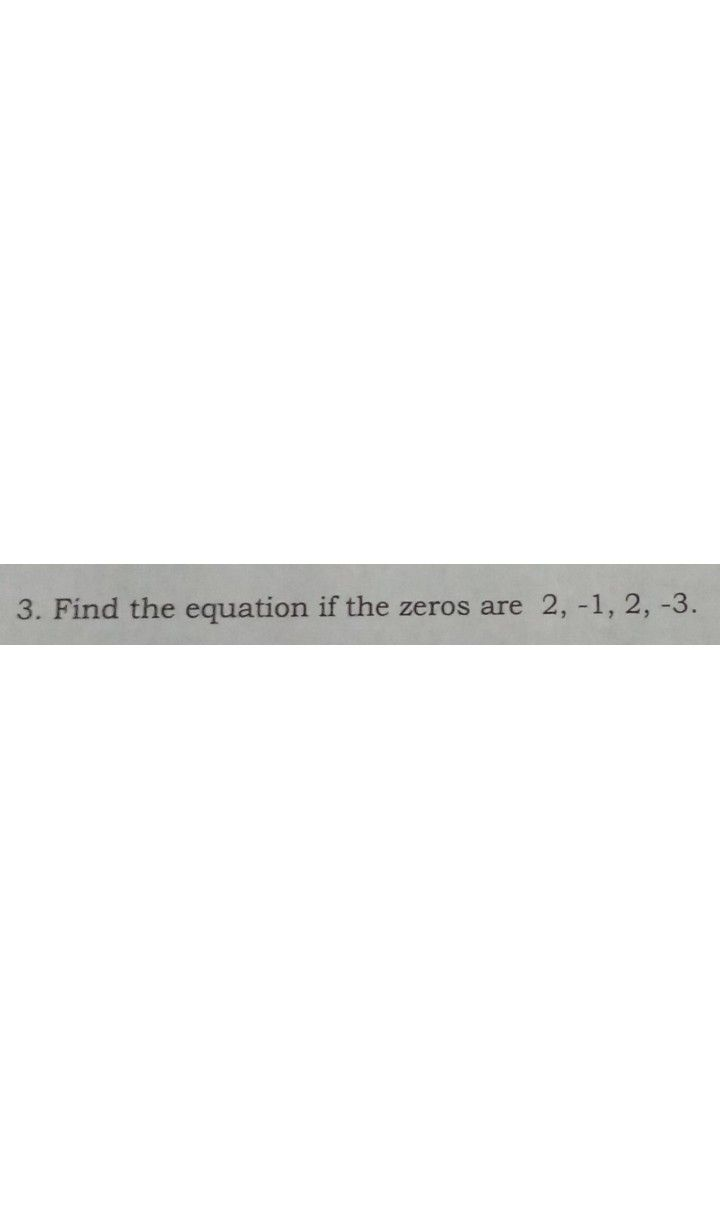Symbol
Problem$1$ 1. Find the roots $of$ $\left(2x+1\right)\left(x+1\right)\left(x-1\right)=0$ $2$ Find the zeros of the polynomial equation $ionx^{4+2x^{3-13x^{2-14x+24}}}=0$ $3$ Find the polynomial equation whose zeros are $e2$ of multiplicity $2,1and-2$ $3$ Find the equation if the zeros are $2,-1,2,-3$
10th-13th grade
Calculus
Search count: 115
SolutionQanda teacher - AskhinaStudent
ok